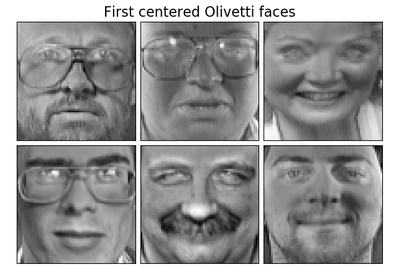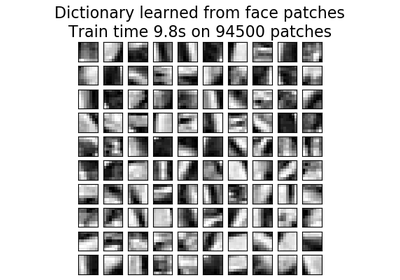# `sklearn.decomposition`.MiniBatchDictionaryLearning¶

class `sklearn.decomposition.``MiniBatchDictionaryLearning`(n_components=None, alpha=1, n_iter=1000, fit_algorithm=’lars’, n_jobs=1, batch_size=3, shuffle=True, dict_init=None, transform_algorithm=’omp’, transform_n_nonzero_coefs=None, transform_alpha=None, verbose=False, split_sign=False, random_state=None)[source]

Mini-batch dictionary learning

Finds a dictionary (a set of atoms) that can best be used to represent data using a sparse code.

Solves the optimization problem:

```(U^*,V^*) = argmin 0.5 || Y - U V ||_2^2 + alpha * || U ||_1
(U,V)
with || V_k ||_2 = 1 for all  0 <= k < n_components
```

Read more in the User Guide.

Parameters: n_components : int, number of dictionary elements to extract alpha : float, sparsity controlling parameter n_iter : int, total number of iterations to perform fit_algorithm : {‘lars’, ‘cd’} lars: uses the least angle regression method to solve the lasso problem (linear_model.lars_path) cd: uses the coordinate descent method to compute the Lasso solution (linear_model.Lasso). Lars will be faster if the estimated components are sparse. n_jobs : int, number of parallel jobs to run batch_size : int, number of samples in each mini-batch shuffle : bool, whether to shuffle the samples before forming batches dict_init : array of shape (n_components, n_features), initial value of the dictionary for warm restart scenarios transform_algorithm : {‘lasso_lars’, ‘lasso_cd’, ‘lars’, ‘omp’, ‘threshold’} Algorithm used to transform the data. lars: uses the least angle regression method (linear_model.lars_path) lasso_lars: uses Lars to compute the Lasso solution lasso_cd: uses the coordinate descent method to compute the Lasso solution (linear_model.Lasso). lasso_lars will be faster if the estimated components are sparse. omp: uses orthogonal matching pursuit to estimate the sparse solution threshold: squashes to zero all coefficients less than alpha from the projection dictionary * X’ transform_n_nonzero_coefs : int, `0.1 * n_features` by default Number of nonzero coefficients to target in each column of the solution. This is only used by algorithm=’lars’ and algorithm=’omp’ and is overridden by alpha in the omp case. transform_alpha : float, 1. by default If algorithm=’lasso_lars’ or algorithm=’lasso_cd’, alpha is the penalty applied to the L1 norm. If algorithm=’threshold’, alpha is the absolute value of the threshold below which coefficients will be squashed to zero. If algorithm=’omp’, alpha is the tolerance parameter: the value of the reconstruction error targeted. In this case, it overrides n_nonzero_coefs. verbose : bool, optional (default: False) To control the verbosity of the procedure. split_sign : bool, False by default Whether to split the sparse feature vector into the concatenation of its negative part and its positive part. This can improve the performance of downstream classifiers. random_state : int, RandomState instance or None, optional (default=None) If int, random_state is the seed used by the random number generator; If RandomState instance, random_state is the random number generator; If None, the random number generator is the RandomState instance used by np.random. components_ : array, [n_components, n_features] components extracted from the data inner_stats_ : tuple of (A, B) ndarrays Internal sufficient statistics that are kept by the algorithm. Keeping them is useful in online settings, to avoid loosing the history of the evolution, but they shouldn’t have any use for the end user. A (n_components, n_components) is the dictionary covariance matrix. B (n_features, n_components) is the data approximation matrix n_iter_ : int Number of iterations run.

Notes

References:

J. Mairal, F. Bach, J. Ponce, G. Sapiro, 2009: Online dictionary learning for sparse coding (http://www.di.ens.fr/sierra/pdfs/icml09.pdf)

Methods

 `fit`(X[, y]) Fit the model from data in X. `fit_transform`(X[, y]) Fit to data, then transform it. `get_params`([deep]) Get parameters for this estimator. `partial_fit`(X[, y, iter_offset]) Updates the model using the data in X as a mini-batch. `set_params`(**params) Set the parameters of this estimator. `transform`(X) Encode the data as a sparse combination of the dictionary atoms.
`__init__`(n_components=None, alpha=1, n_iter=1000, fit_algorithm=’lars’, n_jobs=1, batch_size=3, shuffle=True, dict_init=None, transform_algorithm=’omp’, transform_n_nonzero_coefs=None, transform_alpha=None, verbose=False, split_sign=False, random_state=None)[source]
`fit`(X, y=None)[source]

Fit the model from data in X.

Parameters: X : array-like, shape (n_samples, n_features) Training vector, where n_samples in the number of samples and n_features is the number of features. y : Ignored. self : object Returns the instance itself.
`fit_transform`(X, y=None, **fit_params)[source]

Fit to data, then transform it.

Fits transformer to X and y with optional parameters fit_params and returns a transformed version of X.

Parameters: X : numpy array of shape [n_samples, n_features] Training set. y : numpy array of shape [n_samples] Target values. X_new : numpy array of shape [n_samples, n_features_new] Transformed array.
`get_params`(deep=True)[source]

Get parameters for this estimator.

Parameters: deep : boolean, optional If True, will return the parameters for this estimator and contained subobjects that are estimators. params : mapping of string to any Parameter names mapped to their values.
`partial_fit`(X, y=None, iter_offset=None)[source]

Updates the model using the data in X as a mini-batch.

Parameters: X : array-like, shape (n_samples, n_features) Training vector, where n_samples in the number of samples and n_features is the number of features. y : Ignored. iter_offset : integer, optional The number of iteration on data batches that has been performed before this call to partial_fit. This is optional: if no number is passed, the memory of the object is used. self : object Returns the instance itself.
`set_params`(**params)[source]

Set the parameters of this estimator.

The method works on simple estimators as well as on nested objects (such as pipelines). The latter have parameters of the form `<component>__<parameter>` so that it’s possible to update each component of a nested object.

Returns: self :
`transform`(X)[source]

Encode the data as a sparse combination of the dictionary atoms.

Coding method is determined by the object parameter transform_algorithm.

Parameters: X : array of shape (n_samples, n_features) Test data to be transformed, must have the same number of features as the data used to train the model. X_new : array, shape (n_samples, n_components) Transformed data

## Examples using `sklearn.decomposition.MiniBatchDictionaryLearning`¶Faces dataset decompositionsImage denoising using dictionary learning